An Introduction to One Dimensional Physics and Luttinger Liquid

Cheng-Chien Chen June 7, 2007

(Submitted as coursework for Applied Physics 273, Stanford University, Spring 2007)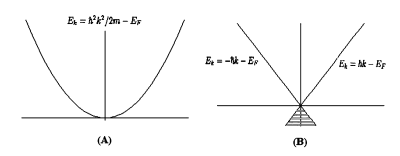Fig. 1: Energy dispersion relations. (A)For free electrons we don't have to worry about writing the Hamiltonian seperately for the right- and left-going fermions because the form is the same for positive and negative k. (B) When we linearize (with the fermi velocity vF set to be 1) the dispersion relation now different for positive and negative k. The energy levels with negative energy are assumed to be filled, as appropriate for a Dirac sea.

The purpose of reporting physics in one-dimension (1D) is twofold: First of all, in 1D the mathematical models are simpler than their higher dimensional counterparts, and more often than not one can has access to exact solutions. Even in 1D the physics is very rich and complicated! Secondly, nowadays there are a number of real systems, such as carbon nanotube, quantum wire, organic superconductors, etc., that should be described by 1D models. Thus 1D physics is no longer artificial but of great concern to both theorists and experimentalists. The content here is mainly based on Ref.1, and briefer introduction to this subject can be found in ordinary many-body textbooks[2,3].

J. M. Luttinger in 1960s discussed an exactly solvable model of a 1D fermionic system. For this reason 1D interacting fermions now bear the name Luttinger liquid. Because of the 1D nature, an electron cannot propogate without pushing its neighbors. No individual motion is possible. Unlike the Fermi liquid, where the quasiparticle is well defined, the low-energy excitation of interacting fermions in 1D are collective. Recall that when collective motion (such as plasma oscillation) occurs, the equation of motion for the density fluctuation resembles that of a harmonic oscillator, which obeys Bose-Einstein statistics. This suggests that we must treat the problem with bosonic fields. This procedure is known as Bosonization.

In this report I shall introduce the technique of Bosonization, which is one of the most important tools for 1D systems, and then discuss some interesting features of Luttinger liquid. In addition, I will address the possibility of 1D superconductivity (since we have learned superconductivity for almost a whole quarter). A few theoretical papers in the 1960s concluded that there should be no superconductive phase in 1D [5,6], yet experiments told us that we do have organic superconductors which can be regarded as 1D systems. Their conclusion is incorrect, and I will try to explain what's the problem with their arguments.

Bosonization

For a 1D electron gas, the excitaion energy of a particle-hole pair is proportional to q, the momentum trasnfer, in the vicinity of the fermi level: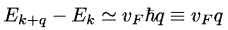The dispersion relation can be linearized as long as q is small compared to the fermi momentum kF. If we use the linear dispersion relation, we are forced to consider the right- and left- going fermions seperately (see Fig. 1). Therefore, the Hamiltonian of the kinetic energy part becomes (for simplicity, we consider spinless fermions first):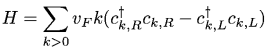where R and L represent right- and left-going particles, respectively.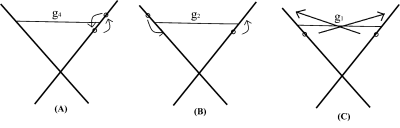Fig. 2: Decomposition of the low energy excitation into three different types (g-ology). (A) g4 couples fermions on the same branch. (B) g2 represents forward scattering. (C) g1 stands for backscattering in which fermions exchange sides.

We now have the kinetic energy part and need the interaction part (for spinless fermions). A typical interaction is: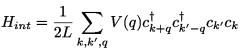where L is the length for the system of interest.

We can decompose the interaction into three different types as shown in Fig. 2. For identical spinless fermions, g2 and g1 processes are the same. The use of notation g to distinguish different processes is historical and called "g-ology".

Historically, the first attempt to solve the full Hamiltonian was made using the fermionic language, which is complicated because the appearance of 4-fermionic operators. However, as mentioned at the beginning, density flucuations (bosonic fields) are a natural basis to use because of the collective tendencies in 1D: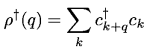The density operator is bosonic, as follows from the anticommutation relations of fermion operators. Let us then write the density operator as some linear combination of bq and bq+, where bq and bq+ are boson annihilation and creaion operators, respectively. Written in the boson basis, the interacting Hamiltonian then becomes: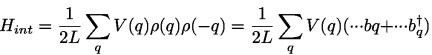This Hamiltonian is quadratic and thus easier to diagonize.

It can be shown that the mapping from all fermionic operators into the bosonic basis is complete. One can then look up the "Bosonization dictionary" to rewrite Hamiltonian in the boson basis. The kinetic energy of the resultant Hamiltonian is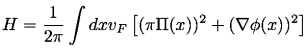where the parameters inside the integrand are defined as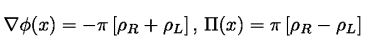Note that since we have introduced an infinite number of occupied states, the Dirac sea, we have to be careful in defining the density operator to avoid infinities .

Features of Lutiinger liquid

If we calculate the g4 process, we will find its contibution to be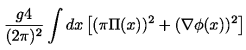which is proportionl to the free Hamiltonian. Therefore, the g4 process only serves to renormalize the velocity of excitations, which becomes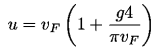Similarly, if we include the calculation for the g2 process, the total Hamiltonian then becomes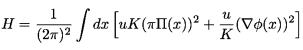which is still quadratic. The parameters u and K are defined as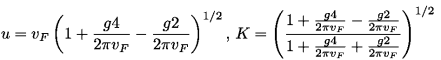Therefore, dealing with the 1D interacting problem in the language of Bosonization is no more complicated than solving the free Hamiltonian.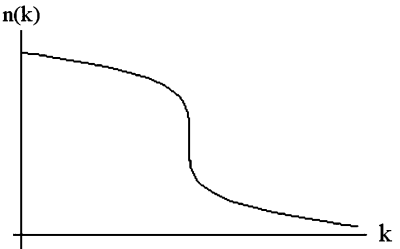Fig. 3:The distribution function in momentum space.

Now we readily see one interesting feature of Luttinger liquid: the velocity of the excititaion u is being renormalized with the presence of the interactions g4 and g2. In general, for fermions with spin, the interactions involving charge and spin are different, so two distinctive excitation velolcities appear. This is known as the spin charge seperation.

Another interesting feature of Luttinger liquid is the occupation number in momentum space. This was first discussed by Luttinger and later refined by Mattis and Lieb. As can be seen in Fig. 3, instead of a discontinuity at kF -the signature of quasiparticle excitation in Fermi liquid - one finds in 1D an essential singularity at kF (the point with infinite slope).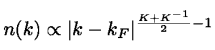In the Fermi liquid theory, one decomposes the spectral function A into a sharp excitation with peak value Z and a smooth background. The distribution in Fig. 3 then means that no individual fermionic excitation can survive in 1D, for this corresponds to Z=0 in Fermi liquid theory. Note that while the shape of the distribution function may be largely distorted, the singularity is still at kF, thanks to the conservation law known as Luttinger theorem: Although the shape of the Fermi surface can be affected by interactions, the "volume" enclosed by the Fermi surface is an invariant. In fact, some of the most convincing observations of Luttinger liquid behavior lie on the measurements in the tunneling density of states. Also, as pointed out by Luttinger, though the distribuion function in momentum space is altered because of interactions, some behaviors near the fermi level (for example, the Kohn effect, a singularity in (q-2kF) remain unchanged.

Superconductivity and phase transition in one dimension

In statistical mechanics it is known from studies of the 1D Ising model that no magnetic ordering exists at finite temperature. (The exception is when there are long-range interactions, which can change the correlation functions qualitatively.) More generally, no phase exhibiting long-range order (LRO) can exist in 1D if only short-range forces exist. Since both the BCS Hamiltonian or the actual physical system involve forces only of finite range, it is then argued that there should be no superconducting phase in 1D. Even if one takes into account modification to BCS by including the compressional mode, it has then be shown by R. Ferrell that these compressional modes, which is more dominant in 1D, prevent the establishment of LRO, which is required for superconductive phenomena. The calculation involves the known theorem that there is no long-range lattice order for 1D geometry. D. Mattis and E. Lieb also showed that in 1D no phase transition at any finite temperature is found based on their model, which proved more rigorously Ferrell's argument.

Their arguments appear to be convincing, yet in reality we do have 1D superconductivity. The flaw of the above argument lies in its assumption that a nonvanishing value of the correlation function G(x-x') for large (x-x') is required by superconducting phenomenon. In other words, LRO isn't required for superconductivity. There is still a critial temperature below which one can tell the difference between an exponential decay and a power law decay for the correlation function. Therefore, there is still a (superconductive) phase even though there is no LRO in the correlation fucntion. However, for a 1D system, if you are sure that there are no long-range interactions and you see a phase with LRO, you probably made a mistake in your experiment (for example, you didn't wait long enough for the system to come to equilibrium).

© 2007 Cheng-Chien Chen. The author grants permission to copy, distribute and display this work in unaltered form, with attribution to the author, for noncommercial purposes only. All other rights, including commercial rights, are reserved to the author.

References

 Thierry Giamarchi, Quantum Physics in One Dimension (Oxford, 2003).

 H. Bruus and K. Flensberg, Many-Body Quantum Theory in Condensed Matter Physics, (Oxford, 2004).

 P. Phillips, Advanced Solid State Physics (Westview Press, 2003).

 J. M. Lutiinger, J. Math Phys. 4, 1154 (1963).

 R. A. Ferrell, Phys. Rev. Lett. 13, 330 (1964).

 D. Mattis and E. Lieb, J. Math Phys. 6, 304 (1965).

 L. D. Landau and E. M. Lifshitz, Statistical Physics, 3rd Ed. Part 1 (Elsevier, 1980).

 C. N. Yang, Rev. Mod. Phys. 34, 694 (1962).